# RD Sharma Solutions for Class 11 Chapter 3 - Functions Exercise 3.1

In Exercise 3.1 of Chapter 3, we shall discuss problems based on function as a special kind of relation, function as a correspondence and equal functions. Students who wish to strengthen their knowledge and have a strong grip over the subject can refer to RD Sharma Class 11 Solutions. Solutions are designed in a simple language by our experts to meet the needs of all students. Here, the solutions to this exercise are provided in pdf format, which can be downloaded easily from the links given below.

## Download the Pdf of RD Sharma Solutions for Class 11 Maths Exercise 3.1 Chapter 3 – Functions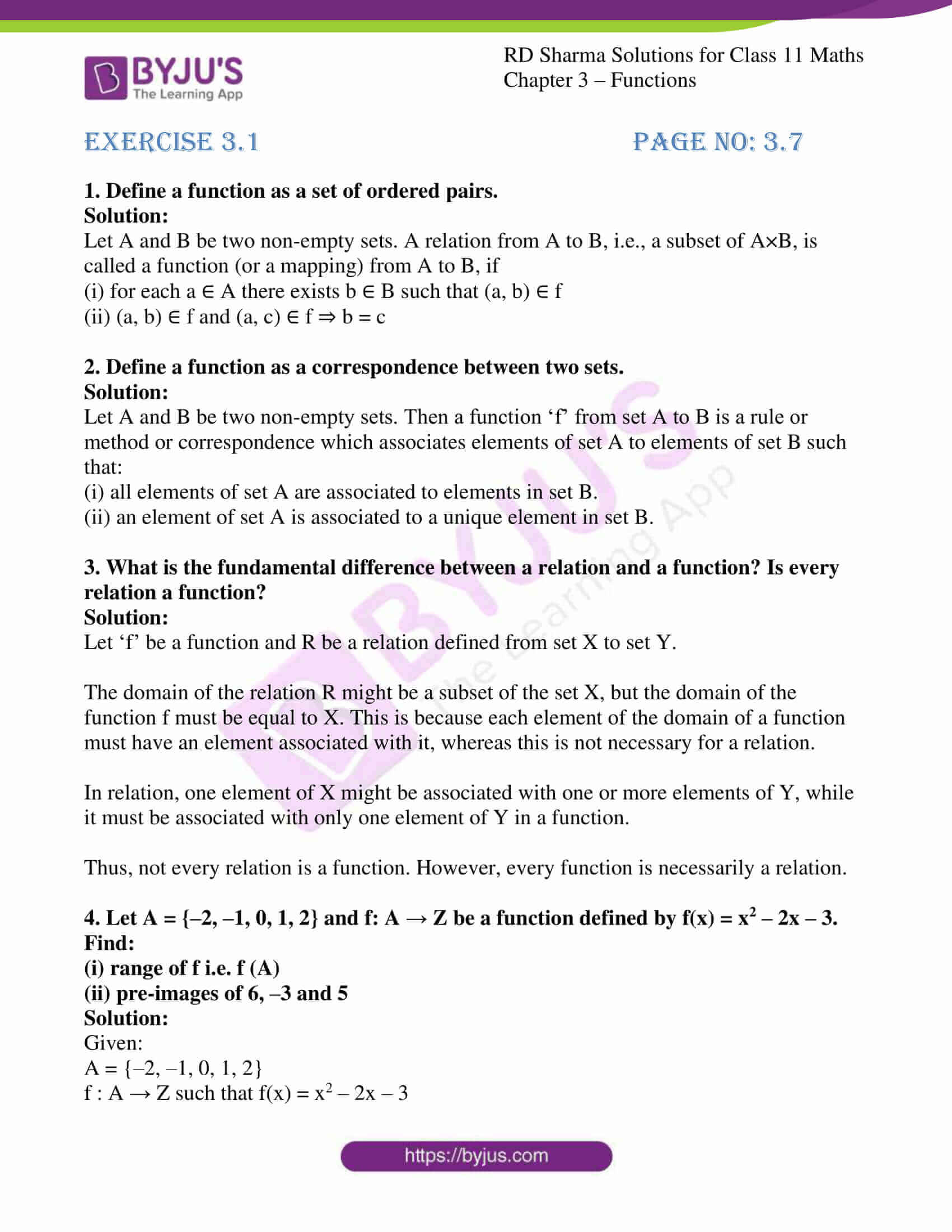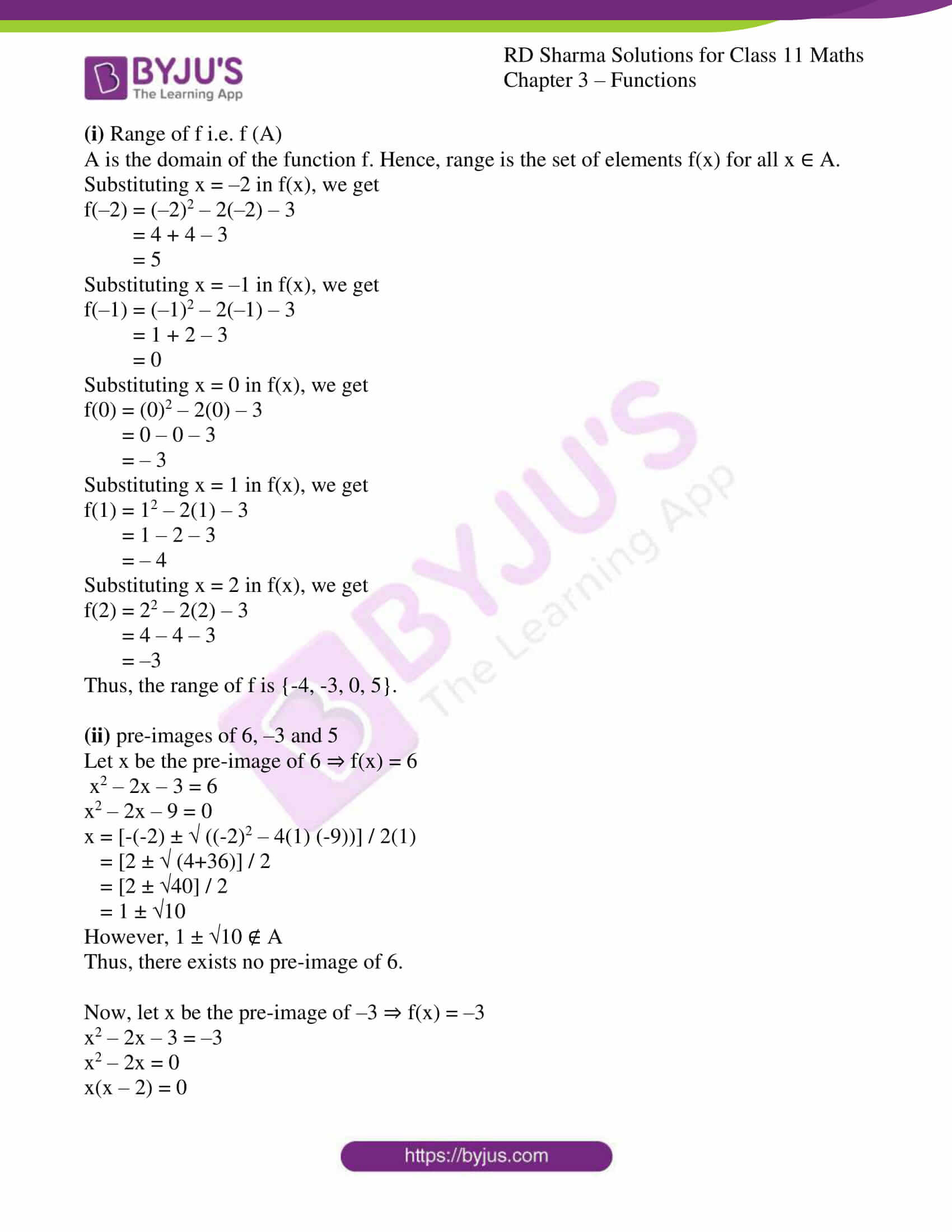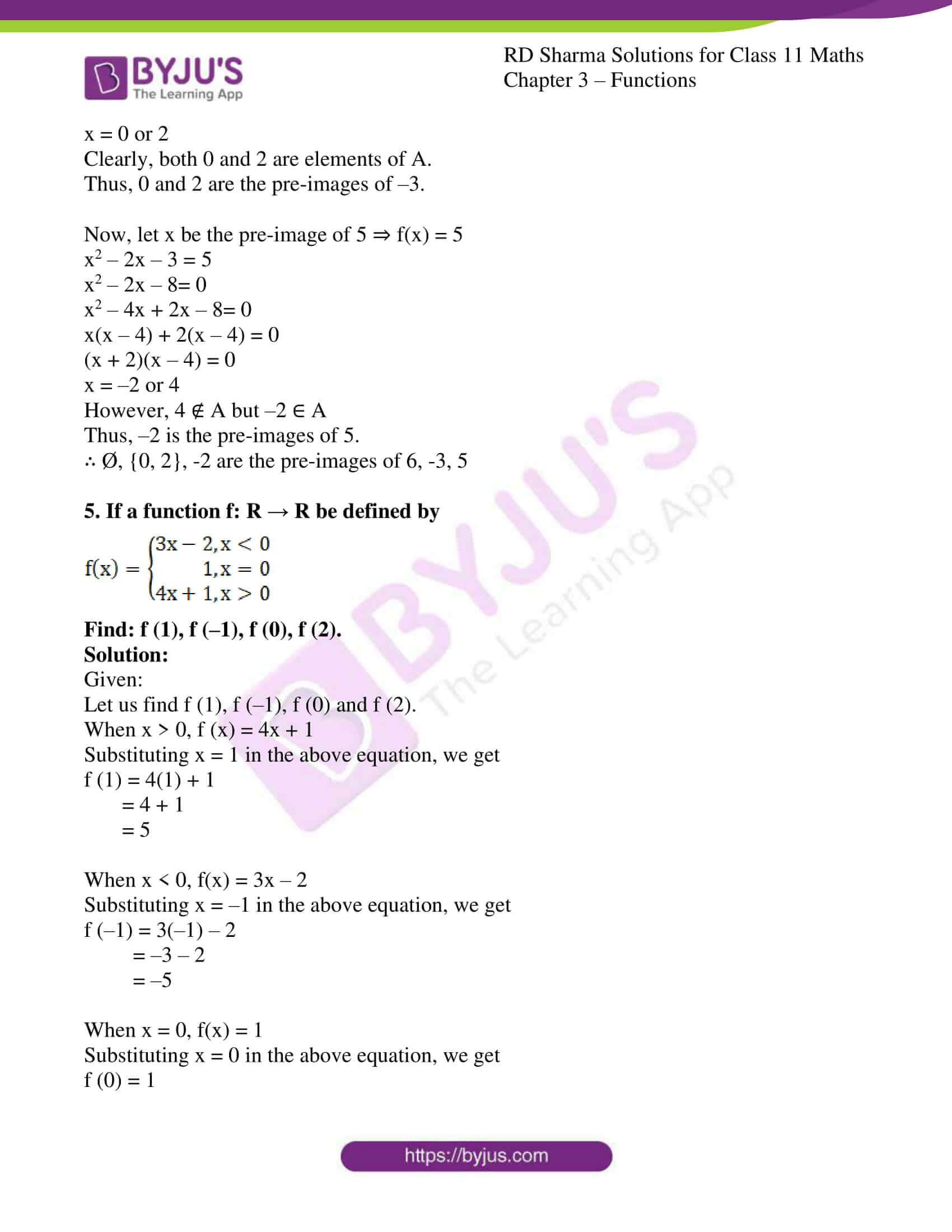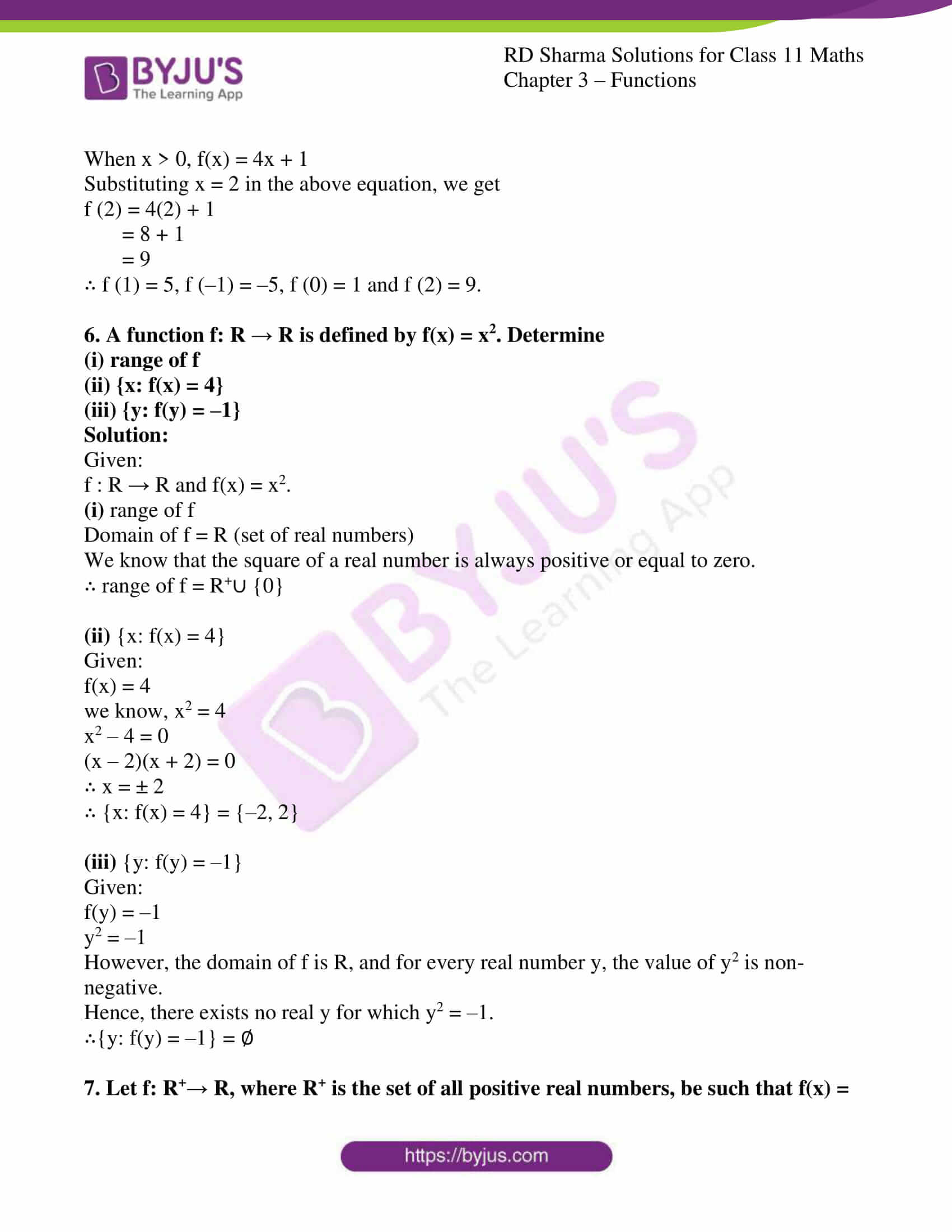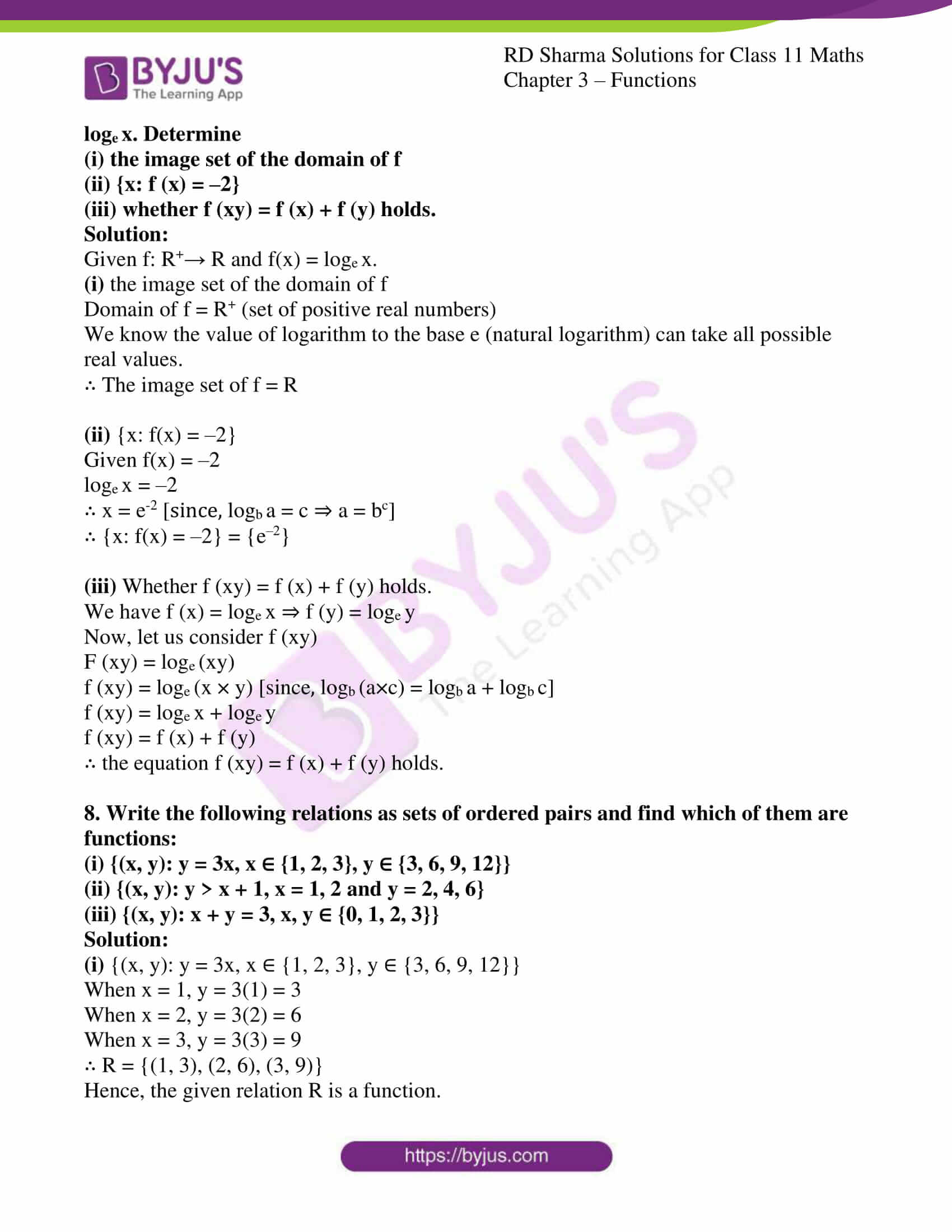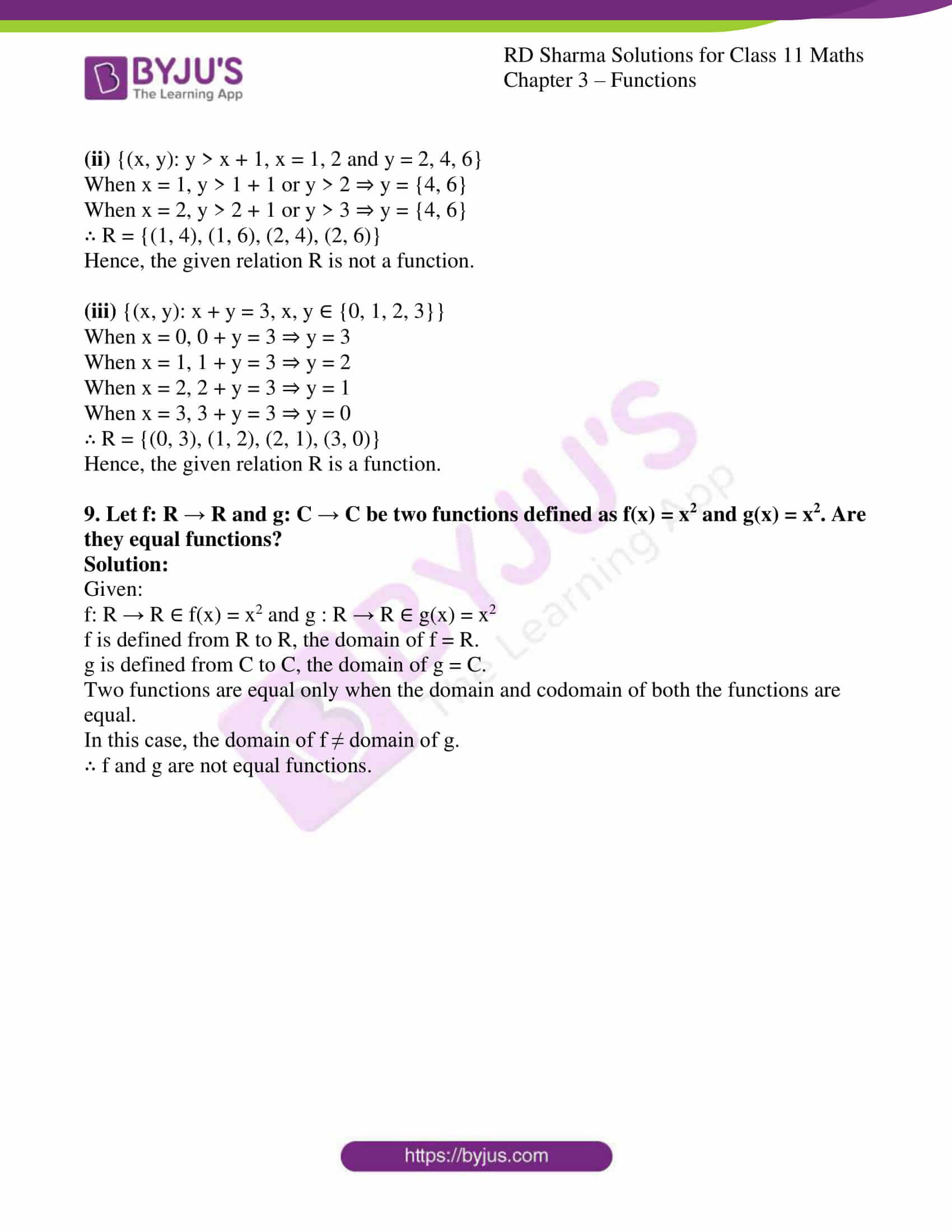### Access answers to RD Sharma Solutions for Class 11 Maths Exercise 3.1 Chapter 3 – Functions

1. Define a function as a set of ordered pairs.

Solution:

Let A and B be two non-empty sets. A relation from A to B, i.e., a subset of A×B, is called a function (or a mapping) from A to B, if

(i) for each a ∈ A there exists b ∈ B such that (a, b) ∈ f

(ii) (a, b) ∈ f and (a, c) ∈ f ⇒ b = c

2. Define a function as a correspondence between two sets.

Solution:

Let A and B be two non-empty sets. Then a function ‘f’ from set A to B is a rule or method or correspondence which associates elements of set A to elements of set B such that:

(i) all elements of set A are associated to elements in set B.

(ii) an element of set A is associated to a unique element in set B.

3. What is the fundamental difference between a relation and a function? Is every relation a function?

Solution:

Let ‘f’ be a function and R be a relation defined from set X to set Y.

The domain of the relation R might be a subset of the set X, but the domain of the function f must be equal to X. This is because each element of the domain of a function must have an element associated with it, whereas this is not necessary for a relation.

In relation, one element of X might be associated with one or more elements of Y, while it must be associated with only one element of Y in a function.

Thus, not every relation is a function. However, every function is necessarily a relation.

4. Let A = {–2, –1, 0, 1, 2} and f: A → Z be a function defined by f(x) = x2 – 2x – 3. Find:
(i) range of f i.e. f (A)
(ii) pre-images of 6, –3 and 5

Solution:

Given:

A = {–2, –1, 0, 1, 2}

f : A → Z such that f(x) = x2 – 2x – 3

(i) Range of f i.e. f (A)

A is the domain of the function f. Hence, range is the set of elements f(x) for all x ∈ A.

Substituting x = –2 in f(x), we get

f(–2) = (–2)2 – 2(–2) – 3

= 4 + 4 – 3

= 5

Substituting x = –1 in f(x), we get

f(–1) = (–1)2 – 2(–1) – 3

= 1 + 2 – 3

= 0

Substituting x = 0 in f(x), we get

f(0) = (0)2 – 2(0) – 3

= 0 – 0 – 3

= – 3

Substituting x = 1 in f(x), we get

f(1) = 12 – 2(1) – 3

= 1 – 2 – 3

= – 4

Substituting x = 2 in f(x), we get

f(2) = 22 – 2(2) – 3

= 4 – 4 – 3

= –3

Thus, the range of f is {-4, -3, 0, 5}.

(ii) pre-images of 6, –3 and 5

Let x be the pre-image of 6 ⇒ f(x) = 6

x2 – 2x – 3 = 6

x2 – 2x – 9 = 0

x = [-(-2) ± ((-2)2 – 4(1) (-9))] / 2(1)

= [2 ± (4+36)] / 2

= [2 ± 40] / 2

= 1 ± 10

However, 1 ± 10 ∉ A

Thus, there exists no pre-image of 6.

Now, let x be the pre-image of –3 ⇒ f(x) = –3

x2 – 2x – 3 = –3

x2 – 2x = 0

x(x – 2) = 0

x = 0 or 2

Clearly, both 0 and 2 are elements of A.

Thus, 0 and 2 are the pre-images of –3.

Now, let x be the pre-image of 5 ⇒ f(x) = 5

x2 – 2x – 3 = 5

x2 – 2x – 8= 0

x2 – 4x + 2x – 8= 0

x(x – 4) + 2(x – 4) = 0

(x + 2)(x – 4) = 0

x = –2 or 4

However, 4 ∉ A but –2 ∈ A

Thus, –2 is the pre-images of 5.

∴ Ø, {0, 2}, -2 are the pre-images of 6, -3, 5

5. If a function f: R → R be defined by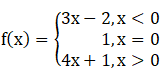Find: f (1), f (–1), f (0), f (2).

Solution:

Given:

Let us find f (1), f (–1), f (0) and f (2).

When x > 0, f (x) = 4x + 1

Substituting x = 1 in the above equation, we get

f (1) = 4(1) + 1

= 4 + 1

= 5

When x < 0, f(x) = 3x – 2

Substituting x = –1 in the above equation, we get

f (–1) = 3(–1) – 2

= –3 – 2

= –5

When x = 0, f(x) = 1

Substituting x = 0 in the above equation, we get

f (0) = 1

When x > 0, f(x) = 4x + 1

Substituting x = 2 in the above equation, we get

f (2) = 4(2) + 1

= 8 + 1

= 9

∴ f (1) = 5, f (–1) = –5, f (0) = 1 and f (2) = 9.

6. A function f: R → R is defined by f(x) = x2. Determine
(i) range of f
(ii) {x: f(x) = 4}
(iii) {y: f(y) = –1}

Solution:

Given:

f : R → R and f(x) = x2.

(i) range of f

Domain of f = R (set of real numbers)

We know that the square of a real number is always positive or equal to zero.

∴ range of f = R+∪ {0}

(ii) {x: f(x) = 4}

Given:

f(x) = 4

we know, x2 = 4

x2 – 4 = 0

(x – 2)(x + 2) = 0

∴ x = ± 2

∴ {x: f(x) = 4} = {–2, 2}

(iii) {y: f(y) = –1}

Given:

f(y) = –1

y2 = –1

However, the domain of f is R, and for every real number y, the value of y2 is non-negative.

Hence, there exists no real y for which y2 = –1.

∴{y: f(y) = –1} = ∅

7. Let f: R+→ R, where R+ is the set of all positive real numbers, be such that f(x) = loge x. Determine
(i) the image set of the domain of f
(ii) {x: f (x) = –2}
(iii) whether f (xy) = f (x) + f (y) holds.

Solution:

Given f: R+→ R and f(x) = loge x.

(i) the image set of the domain of f

Domain of f = R+ (set of positive real numbers)

We know the value of logarithm to the base e (natural logarithm) can take all possible real values.

∴ The image set of f = R

(ii) {x: f(x) = –2}

Given f(x) = –2

loge x = –2

∴ x = e-2 [since, logb a = c ⇒ a = bc]

∴ {x: f(x) = –2} = {e–2}

(iii) Whether f (xy) = f (x) + f (y) holds.

We have f (x) = loge x ⇒ f (y) = loge y

Now, let us consider f (xy)

F (xy) = loge (xy)

f (xy) = loge (x × y) [since, logb (a×c) = logb a + logb c]

f (xy) = loge x + loge y

f (xy) = f (x) + f (y)

∴ the equation f (xy) = f (x) + f (y) holds.

8. Write the following relations as sets of ordered pairs and find which of them are functions:
(i) {(x, y): y = 3x, x ∈ {1, 2, 3}, y ∈ {3, 6, 9, 12}}
(ii) {(x, y): y > x + 1, x = 1, 2 and y = 2, 4, 6}
(iii) {(x, y): x + y = 3, x, y ∈ {0, 1, 2, 3}}

Solution:

(i) {(x, y): y = 3x, x ∈ {1, 2, 3}, y ∈ {3, 6, 9, 12}}

When x = 1, y = 3(1) = 3

When x = 2, y = 3(2) = 6

When x = 3, y = 3(3) = 9

∴ R = {(1, 3), (2, 6), (3, 9)}

Hence, the given relation R is a function.

(ii) {(x, y): y > x + 1, x = 1, 2 and y = 2, 4, 6}

When x = 1, y > 1 + 1 or y > 2 ⇒ y = {4, 6}

When x = 2, y > 2 + 1 or y > 3 ⇒ y = {4, 6}

∴ R = {(1, 4), (1, 6), (2, 4), (2, 6)}

Hence, the given relation R is not a function.

(iii) {(x, y): x + y = 3, x, y ∈ {0, 1, 2, 3}}

When x = 0, 0 + y = 3 ⇒ y = 3

When x = 1, 1 + y = 3 ⇒ y = 2

When x = 2, 2 + y = 3 ⇒ y = 1

When x = 3, 3 + y = 3 ⇒ y = 0

∴ R = {(0, 3), (1, 2), (2, 1), (3, 0)}

Hence, the given relation R is a function.

9. Let f: R → R and g: C → C be two functions defined as f(x) = x2 and g(x) = x2. Are they equal functions?

Solution:

Given:

f: R → R ∈ f(x) = x2 and g : R → R ∈ g(x) = x2

f is defined from R to R, the domain of f = R.

g is defined from C to C, the domain of g = C.

Two functions are equal only when the domain and codomain of both the functions are equal.

In this case, the domain of f ≠ domain of g.

∴ f and g are not equal functions.